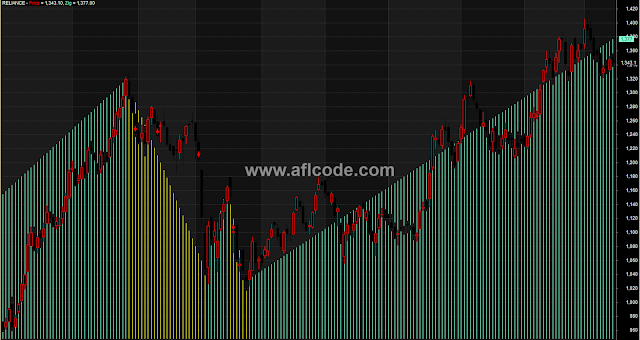### Mother Of All IndicatorMother Of All Indicator

```//www.aflcode.com

AdjBar = Param("Bar",0,0,BarCount,1);
AdjZ = Param("Zig",10,0,20,.1);

//Select Current Tickers OHLC
CO = Ref(O,-AdjBar);
CH = Ref(H,-AdjBar);
CL = Ref(L,-AdjBar);
CC = Ref(C,-AdjBar);

//Select Foriegn OHLC
"Ticker " + TN = Name() ; // Show Ticker
"Index " + BI = GetBaseIndex(); //BI = BaseIndex String
FO = Ref(Foreign( BI,"O"),-AdjBar);
FH = Ref(Foreign( BI,"H"),-AdjBar);
FL = Ref(Foreign( BI,"L"),-AdjBar);
FC = Ref(Foreign( BI,"C"),-AdjBar);

//Create Trend
ZAC = Zig( CC, AdjZ );
UTrend = ZAC > Ref(ZAC, -1);
DTrend = ZAC < Ref(ZAC, -1);

//Do Cross (Create Result Arrays)
t1 = OscP( 3, 6 );
Cond1a= Cross (t1,MACD());
Cond1b= MACD()>-1 AND MACD()<0;
Cond1= Cond1a AND Cond1b;
Cond2 = MACD()>Signal()-.5;
Cond3 = Cross(0,t1);
// Select Conditions to Display.
C1 = Ref(Cond1,-AdjBar);
C2 = Ref(Cond2,-AdjBar);
C3 = Ref(Cond3,-AdjBar);

//Plot Data
Color = IIf(CO > CC, colorBlack, colorRed);
PlotOHLC( CO,CH,CL,CC, "Price", color, styleCandle );//Plot Selected Ticker.
PlotOHLC( FO,FH,FL,FC, BI, colorLightGrey);//Plot Sector Index.
ZColor = IIf(UTrend, colorAqua, colorYellow);
Plot( ZAC, "Zig", ZColor, 3); //Plot ZigZag Trend.

//Show Buy/Sell Arrows.
Buy = C1 AND C2 AND UTrend;
Sell= C3 AND DTrend;
PlotShapes( shapeDownArrow * Sell, colorRed );
PlotShapes( shapeUpArrow * Buy, colorGreen );

// Iterpretation
"Be Aware of ZigZag Limitations.";
"Use CTRL-R to Explore Signals/Zig.";
```

Previous
Next Post »